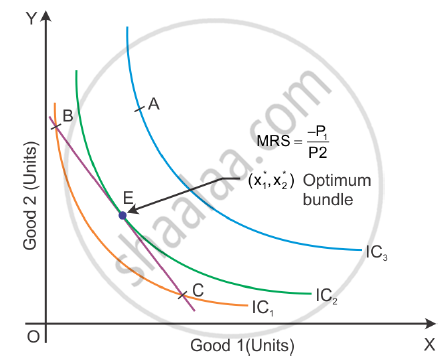Share
Notifications

View all notifications
Books Shortlist
Your shortlist is empty

# Explain the Conditions of Consumer’S Equilibrium Using Indifference Curve Analysis. - CBSE (Arts) Class 12 - Economics

Login
Create free account

Forgot password?

#### Questions

Explain the conditions of consumer’s equilibrium using indifference curve analysis.

Explain the conditions of consumer’s equilibrium under indifference curve approach

Explain the conditions of consumer's equilibrium with the help of the indifference curve analysis.

State the conditions of consumer's equilibrium in the Indifference Curve Analysis and explain the rationale behind these conditions

#### Solution

Conditions of consumer’s equilibrium using indifference curve analysis:

A consumer will strike his equilibrium at the point where the budget line is tangent to an indifference curve.

Slope of IC = Slope of price line

|(-dy)/dx|=|MRS|=|(-P_1)/P_2|

Equality of marginal rate of substitution and ratio of prices: When the budget lines is tangent to an indifference curve at a point, the absolute value of the slope of the indifference curve and of the budget line are equal at that point, i.e. MRS is equal to the price ratio. The slope of the budget line is the rate at which the consumer can substitute one good for the other in the market. At the optimum, the two rates should be the same.
Thus, a point at which the MRS is greater, the price ratio cannot be optimum, and when the MRS is less than the price, the ratio cannot be optimum.

The equilibrium can be represented as follows:In the diagram, Point E shows the consumer’s equilibrium where the budget line is tangent to the indifference curve. Consumers’ desire to purchase correspond to the consumer originally purchase, i.e. x1*, x2* shows the optimum bundle.

Consumer does not reach equilibrium condition at the following points:

At Point B: MRS> - (-P_1)/P_2

At Point A:  MRS> - (-P_1)/P_2

Is there an error in this question or solution?
Solution Explain the Conditions of Consumer’S Equilibrium Using Indifference Curve Analysis. Concept: Indifference Curve.
S